American Journal of Civil Engineering
Volume 3, Issue 4, July 2015, Pages: 107-115

Analysis of Flanged Rectangular Waveguide Probe for Nondestructive Absorbing Materials Characterization Using FDTD Simulation

Department of Electronics and Communications Engineering, Kufa University, Al-Najaf, Iraq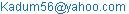Abdulkadhim A. Hasan. Analysis of Flanged Rectangular Waveguide Probe for Nondestructive Absorbing Materials Characterization Using FDTD Simulation. American Journal of Civil Engineering. Vol. 3, No. 4, 2014, pp. 107-115. doi: 10.11648/j.ajce.20150304.13

Abstract: In this paper, the flanged open-ended rectangular waveguide probe technique is studied using Finite Difference Time-Domain simulation (FDTD). Both generally lossy and high loss electromagnetic materials are considered to investigate the influence of probe flange size, operating frequency and sample thickness on complex permittivity (εr) and permeability (μr) and thickness measurement. Variations in the probe flange size for different frequencies, material under test type and thickness are simulated. It is found that using of waveguide probe with finite flange affects probe input reflection coefficient substantially in some cases. To verify the obtained simulations results, a series of experiments are conducted for this purpose. Both εr and μr of material under test under different measurement conditions are extracted using FDTD modeling and compared with reference data. In order to evaluate the degree of accuracy of this technique, error analysis to various sources of errors and most importantly the effect of finite flange size are also demonstrated by using the measured data compared with the analytical model results. Simulations and measurements results have shown that the consideration of probe flange large enough for the practical purpose to be infinite is restricted by the constitutive parameters (εr and μr) and operating frequency as well as the thickness of the material under test. The FDTD simulations and experiments results are presented.

Keywords: FDTD, Numerical Analysis, Error Analysis, Complex Permittivity and Permeability, Rectangular Waveguide, Reflection Coefficient, EM Properties

1. Introduction

The technique using open-ended rectangular waveguide as a probe has its unique advantage for determination of electromagnetic properties of materials. It’s diminishing for some restrictions to sample preparation and openness in structure makes the probe be readily used in nondestructive and in-situ testing. Moreover, waveguide is Powerful once the testing is going to be performed when high absorption material is to be characterized such as radar absorbing materials (RAM) because of high level radiating power from its opened aperture. This technique has attracted many researchers both in theoretical and in technical among others -. As nondestructive testing, most commonly, materials properties are derived from admittance measurement or its equivalent reflection coefficient by inverse problem, where a theoretical formulations for the probe aperture input admittance have been developed for this purpose using analytical procedure. Bakhtiari et al.  examine a conductor-backed material for the purpose of determining the thickness of a lossy dielectric. He uses only the dominant mode to represent the aperture-field distribution. Maode et al.  examine a conductor-backed material with both magnetic and dielectric properties and obtains simultaneous extraction of permittivity and permeability. She employs an approximate variational method to determine the waveguide admittance and hence the reflection coefficient. Stewart et al.  perform parameter extraction of lossy conductor-backed materials using both single and dual-aperture probes. He employs a rigorous, full-wave integral-equation method of analysis and uses the two-thickness method to extract permittivity and permeability using a single-aperture probe.

In the aforementioned theoretical analysis of the probe, the formulations of the aperture input admittance are made based on the assumption that the waveguide opening has infinite conducting flange. Physically, an infinite flange can not be realized in practice. For low loss material characterization, the condition for infinite flange dimension is necessary in order to provide entire interaction between the fields and material under test. In fact , for high-loss materials, the electromagnetic (EM) fields are distributed around the aperture of the sensor over a limited distance consequently, for practical use, the flange with a definite dimension can be used. For this purpose, the flange dimension should be chosen at least as large as to the distance where the decay of field is near negligible to ensure measurement accuracy. The viability of any material characterization method is determined in part by a knowledge of how measurement uncertainty affects the extracted values of εr and μr. For the waveguide probe method, the uncertainty exists in geometrical factors is based on analytical procedure and errors introduced either by the measurement system or in the calculation of the theoretical reflection coefficient . In this regard, the theoretical analysis of electromagnetic models is performed under assumption that the probe has an infinite flange while measurements are usually conducted using a finite-size flange. Consequently, the results of the model and those from measurements may not be sufficiently alike for accurate EM- properties evaluation. Hence, it is necessary in order to obtain a better approximate value in the measurement, the far field of the aperture field distribution should be analyze ; the other way is to calculate the fields of finite flanged aperture directly by using numerical methods. Potentially, the Finite Difference Time Domain technique has several advantages, which make it suitable for modeling complex geometries -. In previous investigations of rectangular waveguide probe, the size of the probe flange is not fully addressed. Many studies, for example,  and , either used the waveguide radiation pattern to determine the proper size of the flange or considered a very large flange. The work presented here investigates the effect of using an open-ended waveguide with finite-sized flange on the input reflection coefficient of rectangular waveguide terminated with the material under test using FDTD modeling under different test conditions. The variation in the flange size for different lossy materials with different thicknesses and frequencies will be studied. Furthermore, a detailed examinations of the effect of using a finite flange are presented and ultimately validated by measuring the complex permittivity and permeability of radar absorbing material samples. Also the error in evaluating both complex permittivity and permeability and thickness is analyzed. Simulation results are to be verified by comparing with published data and measurements to demonstrate the validity of the FDTD technique.

2. Method of Analysis

It is no lost generality to consider the case when the material under is backed by perfect conductor or it may be coated on metal. The geometry of the problem under consideration is shown in Fig.1( a and b). The measurement set-up consists of a flanged waveguide terminated with the material under test. The material under test is considered to be generally lossy or high loss electromagnetic materials with complex permittivity εr and complex permeability μr and thickness d . The analysis of this model is performed using two methods: the analytical method  previously derived based on the assumption that the probe flange is an infinite in extend as shown in Fig.1(a) and the FDTD method. In this paper, the FDTD method is used to model the problem for the case of finite size of probe flange as shown in Fig. 1(b). The object of this analysis is to study the influence of different test conditions such as probe flange size, material type, operating frequency and thickness of material under test on measurement accuracy of complex permittivity and permeability. In the analysis performed, the waveguide used is WR-90 type with square flange of different sizes. The dimensions (a and b) of the waveguide aperture are chosen such that only the dominant TE10 mode propagates within the band of interest (X-band of microwave frequency range).

2.1. Theoretical Modeling for Infinite Flange Case

The geometry of this case of the problem is shown in Fig. 1(a) with Cartesian coordinate system. The flanged open-ended rectangular waveguide probe is in close contact with the flat surface of the material under test. The energy radiated from the probe penetrates through the material under test and reflected back into the aperture. The reflection coefficient (Γ) carries the material properties and thicknesses information.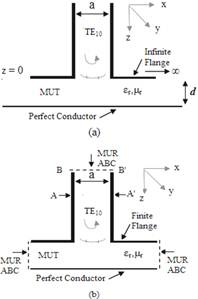Figure 1. Geometry of the problem for (a) Infinite Flange probe (b) Finite Flange probe.

Considering only the dominant TE10 mode, the near-field interaction can be modeled by the following normalized admittance .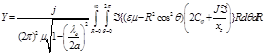(1)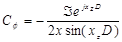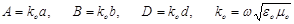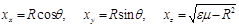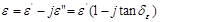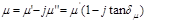where ε, µ are the relative permittivity and permeability, a and b are the wide and narrow dimensions of the rectangular waveguide, and d is the thickness of the material under test. The reflection coefficient is a complicated function of the waveguide dimensions, test frequency (f), sample thickness and the parameters of material under test εr and µr such that Γ(a, b, f, εr, μr, d).

2.2. FDTD Modeling for Finite Flange Case

In this paper FDTD method is employed to model the geometry of the problem with finite flange probe as shown in Fig. 1(b). The main object is to calculate the probe aperture input reflection coefficient under different test conditions since it is quite difficult to use the analytical procedure to formulate aperture input admittance of rectangular waveguide with finite flange. This can be achieved by calculating different field’s components within the probe and material under test and consequently, both complex permittivity and permeability of material under test can be extracted by inverse problem. FDTD method simulates structures in time-domain using a direct form of Maxwell curl equations. In this work, the classical FDTD approach, which is based on Yee’s explicit formulation  is adopted. The model shown in Fig. 1(b) can be considered as combination of 3D cubes in Cartesian coordinates, which are called Yee’s Cells in FDTD method . For an isotropic and homogenous medium with the medium permittivity ε and the medium permeability μ, we have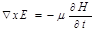(1a)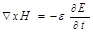(1b))

rearranging these equations yield (2)(2a)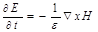(2b)

The FDTD method uses a discretization in time and space (∆t, ∆x, ∆y, and ∆z) to calculate a solution of Maxwell’s curl equations. Evaluating the vector curl operator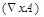and employing central differencing in both time and space to approximate the partial derivatives, we obtain six update equations (one for each component of the electric and magnetic fields). The update equation for the Ex component is: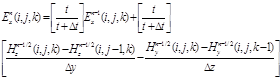(3)

The electromagnetic structure is modeled by approximating its geometry and composition with Yee cells of different material parameters; both permittivity and permeability of material under test and inside of the waveguide. In accordance with FDTD principles, to ensure adequacy and accuracy of computation, the model is meshed with cubic cells chosen so that the cell size is sufficiently smaller than λ in the material under test as well as it is in accordance with the Courant stability criterion . In this work, FDTD available space was divided into many rectangular three dimension cells with the size Δx, Δy and Δz in x, y and z axis respectively. A fine spacing cell with dimensions of Δx =0.05mm, Δy =0.05mm, Δz =0.05 mm is used within the probe and the material under test to increase the accuracy of the calculation. Effective (average) values of constitutive parameters are used to calculate field components at different interfaces. The computational domain is truncated by first-order Mur absorbing boundary condition (ABC)  at plane B-B’ of the waveguide and at the radial boundary of the problem space to limit the computational domain and increase the computational efficiency. An excitation of TE10 mode is then applied at the excitation plane A-A’ to the computational model and the E-field and H-field computations are alternately matched through time from time zero to the desired stopping point. The field distribution in the sample and the probe are calculated using Yee cell procedure. The input admittance of the material under test is obtained by calculating field’s components within material under test. According to the transmission line theory, the probe complex reflection coefficient, Γo, is calculated using (4) at sampling point located away from the aperture to avoid higher order modes that may exist at the aperture layered media interface boundary.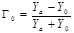(4)

where Ya and Y0 are the aperture admittance and the equivalent characteristic admittance of the waveguide, respectively.

3. FDTD Modeling Validation

A 3D FDTD code is developed to calculate complex reflection coefficient of the probe terminated with the material under test as shown in Fig. 1(b). In order to validate the FDTD code; several simulations and tests were conducted for different flange sizes. Samples of different materials were used to calculate the input reflection coefficient of the probe over X-band (8.2 – 12.4 GHz) of microwave frequency range. Measurement corresponding to the selected cases of the flange sizes was performed using HP-8510B automatic network analyzer (ANA) with WR-90 waveguide probe over the given frequency range. Table I shows a comparison between the results of calculated reflection coefficient using FDTD modeling versus data obtained analytically previously published in . The material under test used is radar absorbing material (RAM) MF 116 with complex permittivity of εr =16 - j0.96 and complex permeability of μr = 1.5 – j1.02 with thickness of 2.44 mm. The results obtained are calculated at frequency of 10 GHz where it may be seen that there is good agreement between them. Table II compares the measured and the calculated magnitudes of the reflection coefficients using FDTD method for selected dimensions of probe flange using the same material under test with thickness of 4.18 mm. The measured variation in the reflection coefficient closely follows the FDTD simulation results validating the computation tool.

4. Error Analysis

Radar absorbing materials are frequently encountered in many applications such as electromagnetic compatibility (EMC) and shielding. The characterization of these materials are usually performed nondestructively using flanged open-ended rectangular waveguide probe[6-8] since this technique is quite suitable for solid materials testing. In this method of measurement, there are many possible sources of error which can be broadly categorized into two groups: those associated with the measurement of the reflection coefficient (measurement error sources) and those associated with computing the theoretical reflection coefficient. The latter includes uncertainty in the modeling of the applicator. Moreover, the flange size is assumed to be an infinite in extend. Using of a waveguide with small flange size will significantly influence measured values and error factors are introduced/increased in the measurement of both complex permittivity and permeability which is one of stated goals of this paper.

Table I. Comparison Between the FDTD Results versus Reference Data  of the Reflection Coefficient.

 Γ (Magnitude) Γ (Phase in Degree) FDTD Model Reference  FDTD Model Reference  0.711 0.699 -68.10 -66.49

Table II. Comparison of the Measured and the Calculated Magnitude of the Reflection coefficient for Different Flange Sizes of the Probe.Flange Size (mm) 40 50 60 Method FDTD Model 0.4358 0.4420 0.4542 Experiment 0.4198 0.4282 0.4481

4.1. Influence on Reflection Coefficient Measurement

To evaluate the effect of probe flange size on reflection coefficient measurement accuracy, a series of simulations are conducted for different flange dimensions. Samples of different materials are used to calculate the input reflection coefficient of the probe over the given frequency range. Different measurement conditions such as operating frequency, variation of constitutive parameters (εr, μr) values of material under test and the thickness of the material under test are considered for this purpose. Fig.2 shows variation of the calculated reflection coefficient of the probe terminated in lossy material with the operating frequency for different sizes of the flange. Two frequencies of 9 and 11 GHz are selected. It is clear from the figure that the variation in the magnitude of reflection coefficient is less at 11 GHz than at 9 GHz. This may be due to increasing of radiation losses at higher frequencies. The influence of constitutive parameters values of two different materials (lossy and high loss) on the measurement is also studied. In Fig. 3, the calculated percent of deviation in reflection coefficient (compared with that of probe with infinite flange size) due to variations in the flange size normalized to the wavelength in the material under test (λm) for this case are plotted. It is clear from the figure that the variation of reflection coefficient with the flange size decreases for high loss material. This is due to that for material with high loss, the fields decay faster than that of low loss materials. Fig. 4 shows the effect of material under test thickness on reflection coefficient measurement. As shown, for thick sample, the effect of flange size on reflection coefficient is less compared to thin sample. This is due to that for absorbing materials, the reflection decreases with increasing of sample thickness. For this reason the sensitivity of the thickness measurement of lossy materials is becoming poorer for thicker lossy material. From the FDTD analysis, according to the calculated probe reflection coefficient for different measurement conditions, it can be concluded that the choice of flange dimension of rectangular waveguide probe for generally lossy and high loss material characterization depends on sample thickness, εr and μr parameters of material under test and the operating frequency. In general, to ensure measurement accuracy, the flange dimension should be chosen at least as large as to the distance where the decay of the deviation of reflection coefficient is negligible and this issue will be further investigated in the following section.Figure 2. Variation of magnitude of reflection coefficient versus flange size at different frequencies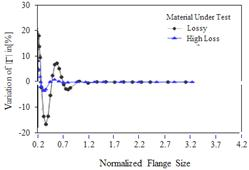Figure 3. Variation of magnitude of refection coefficient in [%] versus flange size normalized to the wave- length in the material under test for two different lossy materials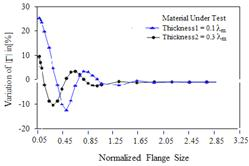Figure 4. Variation of magnitude of refection coefficient in [%] versus flange size normalized to the wave- length in the material under test for two different thicknesses of lossy materials

For the cases examined of the lossy materials, the reflection coefficient had a damped oscillatory dependence on the probe flange size.

4.2. Influence on Constitutive Parameters Measurement

The viability of any material-characterization method is determined in part by a knowledge of how measurement uncertainty affects the extracted values of εr and μr. The sensitivities of εr and μr to the measured values of reflection coefficient must be understood in order to determine the conditions under which a given method may be used effectively. In this section, the analysis performed in the previous section based on FDTD modeling is to be verified experimentally in order to investigate the influence of waveguide flange size on the measured complex permittivity and permeability. Since two complex quantities are to be determined (εr and μr) and the material under test is assumed to be conductor backed, an experimental procedure is required in which two-independent reflection coefficients are measured for a given probe flange size. Since frequency is an independent variable of the probe’s reflection coefficient, which can be symbolized as Γ(a, b, f, ε(f), μ(f ), d ), swept-frequency technique is used to produce needed independent reflections . Several experiments are conducted to investigate the influence of probe flange size and sample thickness on EM-properties measurement accuracy using waveguide probe with different flange dimensions over X-band of microwave frequency range (8.2-12.4) GHz. Table III shows variations of the measured permittivity and permeability (both real part and loss tangent) for three selected flange dimensions of 30 mm, 60 mm and for the case when the flange is an infinite in extend compared with reference data at frequency of 10 GHz. The material under test used is GEC-Marconi 9052 radar absorbing material with thickness of 2.08 mm. The frequency interval Δf used in the measurement is taken to be 0.3 GHz. For cases of 30 mm and 60 mm flange sizes, the results of both permittivity and permeability are obtained using FDTD modeling while for the case when the flange size is assumed to be an infinite in extend, the results are obtained analytically using (1). The reference data are given by the ECCOSORB MF Technical Bulletin and the Marconi Company . It is clear from the table that the data obtained for the cases of 60 mm and infinite flange sizes and reference data are fairly consistent. As expected a large discrepancy is obtained between the measured results for the case of the flange with size of 30 mm (both in real parts and loss tangent) and reference data. This is due to that for a given lossy magnetic material, a 30-mm flange size is not enough to ensure adequate decay of the field resulting in radiated field from the material under test boundary. Furthermore, the edge-diffracted fields at the flange-edge boundary with the material under test produce a spurious reflection especially for the cases of measurement when the material under test is thin or low loss. As the flange size increases, the interaction between the EM fields and material under test increases by permitting a larger field into the material under test resulting improvement in measurement accuracy. Also, one can observe that a small deviation in the measured results of permittivity and permeability for the cases of using waveguide with 60 mm flange size and that obtained analytically under assumption that the flange size is infinite in extend as compared to the reference data. This is due to that for the second case the measurements are performed using standard X-band waveguide fitted with a square flange with side dimensions of 47.8 mm x 47,8 mm while in the theoretical formulation the flange is assumed to be infinite. For the first case, the probe flange size used in the measurement may be adequate to provide accuracy in the measurements in. Consequently, for practical use, the flange with a definite dimension must be used. This is also validated in .

Table III. Comparison Between the Measured Complex permiottivity and permeability of Lossy Material Under test For Different Probe Flange Size. Measurements are performed at f = 10 GHz

 Flange Dimension (mm) Measurement Results er tande mr tandm 30 mm 18.52 0.041 1.95 1.198 60 mm 18.26 0.021 1.76 1.136 Analytical Method  17.94 0.016 1.83 1.084 Reference Data 18.18 0.023 1.8 1.194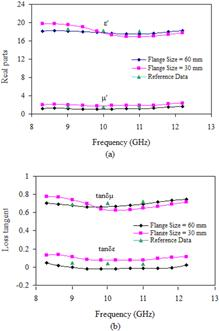Figure 5. Variation of complex permittivity and permeability of radar absorbing material with frequency for different probe flange size

In order to get a better insight into the nature of the problem and estimate the main effective sources of error in the measurement, another set of measurements are performed over a given frequency range to investigate the influence of sample thickness on the extracted εr and μr. The same material under test is used for this purpose with thickness of 6.24 mm. The variations of the permittivity εr and permeability μr (both real parts and loss tangents) as a function of frequency are shown in Fig. 5 (a and b) respectively. Fig.5a shows variations of the real parts of permittivity (ε’) and permeability (μ’) while Fig. 5b shows variations of the loss tangents of permittivity (tanδε) and permeability (tanδμ). It can be seen from the figures that good agreement between the measured results and the reference data is achieved over the given range for the case when the flange size is 60 mm., but the thickness effect on sensitivity and accuracy is obvious especially for complex permittivity extraction . The results in Fig. 5b show a large discrepancy in the loss tangent (especially tanδε) as compared to the reference data. Hence, the sample whose thickness is too thicker are dominant reasons. This is due to that if the thickness is too thick, the variation of reflection coefficient ( both the amplitude and the phase) becomes duller, and finally tends to a point. This is because the sample is so thick that the decaying reflection wave from the short-circuit plate can not influence the input wave on the flange plane. On the other hand, if the thickness is chosen to be too small, the spurious reflection coefficient would become too high especially for low loss material. It would lead to some difficulty in ensuring the testing accuracy. So it is important to choose a proper flange size and thickness for testing. Furthermore, the open-ended waveguide probe is at its best for measuring the high-loss materials such that it is difficult to get reasonable accuracy for tanδ which is less than 0.1 (here, the actual tanδε is about 0.06). The results obtained from this analysis show that generally lossy materials characterization is highly affected by probe flange dimensions, operating frequency and thickness of sample under test. In this work both complex permittivity and permeability of the material under test are extracted by inverse problem using iterative optimization technique from the calculated reflection coefficient (Γc) using FDTD modeling and measured reflection coefficient (Γm). Consequently, an iterative solution are sought to compute εr and μr for given Γcr , μr) and Γmr , μr) with an optimum objective function using (5).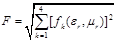(5)

From the results of the FDTD simulations and experiments, the desired solution of εrand μr can be obtained by minimizing the function F. At the beginning, initial values of εrand μr are assumed, then ΓC1 and ΓC2 are calculated from (4) using FDTD modeling and F can be obtained from (5). If F is greater than a desired tolerance ζ , new values will be updated automatically using iterative algorithms such as Newton-Raphson method or levenberg-Marquardt Method . Then a new value F is evaluated from the new εrand μr This procedure is repeated until the value of F is less than ζ . These iterative algorithms guarantee a correct convergence in most cases but the converge process is very time-consuming especially for multiparameters measurement. A computer program is written to implement this optimization procedure. Figure 6 shows the algorithm for calculating both complex permittivity and permeability of material under test using Newton-Raphson method.

5. Evaluation of Error

The results of error analysis performed in the previous section showed that one major source of error in measurement of both complex permittivity and permeability using flanged rectangular waveguide probe can be due to the finite size of the flange and other test conditions. Since the choice of the material under test thickness depends on the parameters εr, μr and the operating frequency, therefore, it is necessary in order to obtain a adequate measurement accuracy, evaluating the percentage error due to the exclusion of finite size of the flange for different measurement conditions. Consequently, a general guidelines can be suggested for measurement set-up geometries. In this section, the evaluation of % error due to using finite flange size is investigated and discussed for the cases of measurement results obtained in the previous section.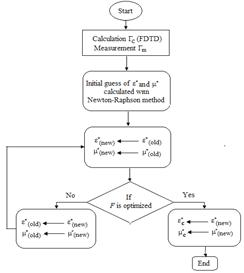Figure 6. Flowchart of calculation of complex permittivity and permeability

The percent error, in real parts and loss tangents of both permittivity and permeability is defined using (6)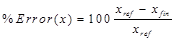(6)

where x is the real part or loss tangent of both complex permittivity and permeability, xref is the reference value of real part and loss tangent of both complex permittivity and permeability of the material under test and xfin is the value of real part and loss tangent of both complex permittivity and permeability measured using finite flange probe. Computation of error is performed by comparing the results of complex permittivity and permeability obtained using FDTD method ( for finite flange size) and the spectral domain analysis method using (1) for the case when the flange is considered to be an infinite in extend with the reference data for various material under test thicknesses of 2.08 mm and 6.24 mm respectively. Tables IV summarizes the calculated values of the % error at operating frequencies of 9 GHz for sample thickness of 2.08 mm while table V summarizes the calculated values of. the % error for sample thickness of 6.24 mm. It is clear from table IV and table V that the percentage error increases with decreasing probe flange size for both real and loss tangent of complex permittivity and permeability and this error decrease as flange size increases. The influence of sample thickness on measurement error is also obvious from the results of the two tables. For thin sample of 2.08 mm, the percent error in the measurement increases especially in the real part of complex permittivity. On the other hand, one can observe a large discrepancy between the measured and the reference data of the loss tangents of both complex permittivity and permeability for sample thickness of 2.08 mm. For case of the sample with 6.24 mm thickness, the calculated % error decreases for measured real parts of both complex permittivity and permeability. As expected, the % error for the loss tangent of complex permittivity (tanδε) increases for the reasons discussed in the previous section. Also the effect of probe dimensions and operating frequency on measurement accuracy is examined. Table VI shows the calculated values of the % error for the sample with thickness of 6.24 mm since this value of sample thickness represents the worst case in the measurement. The calculations are performed at operating frequency of 11GHz with different flange dimensions as illustrated in Fig. 5. and compared with the results of table V. It is clear from the results of the two tables that the % error for the case of 11 GHz is much lower for different flange dimensions than that obtained for the case of 9 GHz. This is reasonable since the radiation increase with increasing the frequency. the results of error analysis performed in this section show that there are three factors influence measurement accuracy when a flanged open-ended rectangular waveguide probe is used for nondestructive testing of generally lossy or high loss materials namely flange size, operating frequency and sample thickness. Among these three factors it is clear that flange size and operating frequency are key factors in measurement since the material under test is assumed to be coated or backed by metal with several millimeter thickness. In this regards, the imaginary part of complex permittivity is affected for thicker sample. From the results obtained, it is difficult to make a specific evaluation regarding using finite flange rectangular waveguide for nondestructive testing of generally loss and high loss materials since there are many factors should be taken into account. In general, from Figs. 2, 3 and 4, it can be concluded that for a thick lossy materials and low values of operating frequency , flange size larger than λm is sufficient for accurately measuring both complex permittivity and permeability and thickness since the error in the measurement caused by the non-infinite flange is very small. However, if the material under test is thin then a relatively large flange is required (larger than 2λm) to have an accurate measurement of εr and μr. Hence, one can design the measurement setup with an adequate flange size to obtain the desired accuracy in the measurement.

Table IV. Estimated values of complex permittivity and permeability of 2.08 mm thickness sample and errors as compared to the reference values of εr =18.64(1 –j0.045) and µr = 1.7(1-j 0.71) at f = 9 GHz.

 Flange Size (mm) Complex permittivity Complex permeability εr = ε’(1 – j tanδε)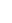μr = μ’(1 – j tanδμ) Real part error [%] tan δε error [%] Real part error [%] tan δμ error [%] 30 -13.76 -52.32 -10.32 -7.32 60 -4.12 -39.62 3.63 -2.56 Infinite -3.71 -45.74 -4.82 -4.15

Table V. Estimated values of complex permittivity and permeability of 6.24 mm thickness sample and errors as compared to the reference values of εr =18.64(1 –j0.045) and µr = 1.7(1-j 0.71) at f = 9 GHz

 Flange Size (mm) Complex permittivity Complex permeability εr = ε’(1 – j tanδε) μr = μ’(1 – j tanδμ) Real part error [%] tan δε error [%] Real part error [%] tan δμ error [%] 30 -6.54 -82.41 -5.84 -11.o6 60 2.56 -64.24 4.10 -4.45 Infinite -1.45 -71.12 -2.21 -6.98

Table VI. Estimated values of complex permittivity and permeability of 6.24 mm thickness sample and errors as compared to the reference values of εr =18.21(1 –j0.042) and µr = 1.62(1-j 0.72) at f = 11 GHz

 Flange Size (mm) Complex permittivity Complex permeability εr = ε’(1 – j tanδε)μr = μ’(1 – j tanδμ) Real part error [%] tan δε error [%] Real part error [%] tan δμ error [%] 30 3.54 -36.43 -5.54 3.88 60 1.54 -26.34 2.10 5.87 Infinite 2.32 -56.21 -1.26 1.46

6. Summery and Conclusions

In our study of using open-ended rectangular waveguide probe system for nondestructive testing, we analyzed the influences of several key parameters of measurement set-up on both complex permittivity and permeability and thickness measurement of generally lossy and high loss materials using FDTD simulation and measurement. The performed analysis has shown that the errors in measurement using this technique are highly affected by probe flange size and operating frequency especially for generally lossy materials. Also uncertainty of the material under test thickness does have some effects on the measured value of high loss materials complex permittivity and permeability, but this parameter is much easier to control, especially for solid samples. Simulation and measurement results with various flange configurations indicated that it is difficult to use the analytical models to include the effect of the finite flange size. In general, for the lossy materials, the material under test and the operating frequency as well as thickness restrict the consideration of flange large enough for the practical purpose to be infinite. By FDTD modeling, the required extend of the flange can be determined. The results presented in this paper may be useful in coating materials nondestructive testing and other applications such as thickness measurement of composite materials.

References

1. E. Nyfors and P. Vainikainen, Industrial Microwave Sensors, Artech House, 1989.
2. M. Hirano, M. Takahashi, and M. Abe, "A study on reflection coefficient from lossy dielectric by using flanged rectangular waveguide," IEICE Trans. Electron. vol. J82-C-I, no.5, pp.283–287, May 1999.
3. Mazlumi, F., S. H. H. Sadeghi, and R. Moini, "Interaction of an open-ended rectangular waveguide probe with an arbitrary- shape surface crack in a lossy conductor," IEEE Trans. Microwave Theory Tech., Vol. 54, No. 10, pp. 3706,-3711, 2006.
4. Hyde IV, M. W., J. W. Stewart, M. J. Havrilla, W. P. Baker, E. J. Rothwell, and D. P. Nyquist, "Nondestructive electromagnetic material characterization using a dual waveguide probe: A full wave solution," Radio Sci., Vol. 44, RS 3013, 2009.
5. S. Bakhtiary, S. I. Ganchev and R. Zoughi, " Open-ended rectangular waveguide for nondestructive thickness measurement and variation detection of lossy dielectric slabs backed by a conducting plate," IEEE Trans. IM, vol. IM-42, pp. 19-42, 1993
6. Maode, N., S. Yong, Y. Jinkui, F. Chrnpung, and X. Deming, "An improved open-ended waveguide measurement technique on parameters εr and μr of high- loss materials," IEEE Trans.Instrum. Meas., Vol. 47, No. 2, pp. 476-481, April 1999.
7. Stewart, J. W. and M. J. Havrilla, "Electromagnetic characterization of a magnetic material using an open- ended waveguide probe and a rigorous full-wave multimode model," in Journal of Electromagnetic Waves and Applications, Vol. 20, No. 14, pp. 2037-2052, 2006.
8. G. D. Dester" Error analysis of a two-layer method for the electromagnetic characterization of conductor- backed absorbing materials using an open-ended waveguide probe" Progress In Electromagnetics Research B, Vol. 26, pp. 1-21, 2010.
9. Abdulkhadim A. Hasan, D. Xu, and Y. J.Zhang" Modeling and analysis of finite flange open-ended coaxial probe for planar and convex surface coating material testing by FDTD-method," Microwave and Optical TechnologyLetters, vol. 24 no. 2, pp. 117- 120, January 2000.
10. K. Shibata O. Hashimoto, and R. K. Pokharel" Analysis of error due to exclusion of higher modes on complex permittivity measurement using waveguide with flange" IEICE Trans. Electron, Vol.E88–C, NO.1 January 2005 pp. 139-142
11. Abdulkhadim A. Hasan, D. Xu, Z. Lin , and N. Maode" A Modified open- ended coaxial probe for concave surface coating materials testing," 2000 IEEE MTTs International Microwave Symposium Digest.
12. F. Kung and H. T. Chuah’ A Finite-Difference Time- Domain (FDTD) software for simulation of printed circuit board (PCB) assembly’ Progress In Electromagnetics Research, PIER 50, pp. 299–335, 2005.
13. C.-W. Chang, K.-U. Chen, and J. Qian, "Nondestructive determination of electromagnetic parameters of dielectric materials at X-band frequencies using a waveguide probe system," IEEE Trans. Instrum. Meas., vol. 46, no. 5, pp. 1084–1092, Oct. 1997.
14. K. S. Yee,"Numerical solution of initial boundary-value problems involving Maxwell’s equations in isotropic media", IEEE Trans Antenna Propag. AP, vol.14. pp. 302-307, 1966.
15. A. Taflove and M. E. Brodwin, " Numerical solution of steady-state electromagnetic scattering problems using the time-dependent Maxwell’s equations, "IEEE Trans, Microwave Theory Tech, vol, MTT-23, pp. 623-630, 1975.
16. G. Mur,"Absorbing boundary condition for the finite- difference approximation of the time-domain electroma- gnetic field equations", IEEE Trans. Eectromag. Compat. EMC-22, pp. 377-382, 1981.
17. M. T. Ghasr, Devin Simms, and R. Zoughi," Multimodal solution for a waveguide radiating into multilayered structures—dielectric property and thickness evaluation" IEEE Trans. Inst & Meas, vol. 58, NO. 5, pp. 1505-1513, May 2009.
18. S. Wang, Abdulkadhim A. Hasan, N. Moade and X. Deming," A Swept-frequency technique with an open-ended waveguide sensor for nondestructive, simultaneous determination of thickness, permittivity and permeability of radar absorbing coatings" 1998 Asia Pacific Microwave Conference, pp 129-132.
19. Emerson & Cuming, Microwave Products, Inc., ECCOSORBR°FGM Permittivity & Permeability Data," 2007
20. W. H. Press, S. A. Teukolsky, W. T. Vetterling and B. P. Flannery, Numerical Receips in C+, second Edition, Cambridge University Press, 1992

 Contents 1. 2. 2.1. 2.2. 3. 4. 4.1. 4.2. 5. 6.
Article ToolsAbstractPDF(372K)